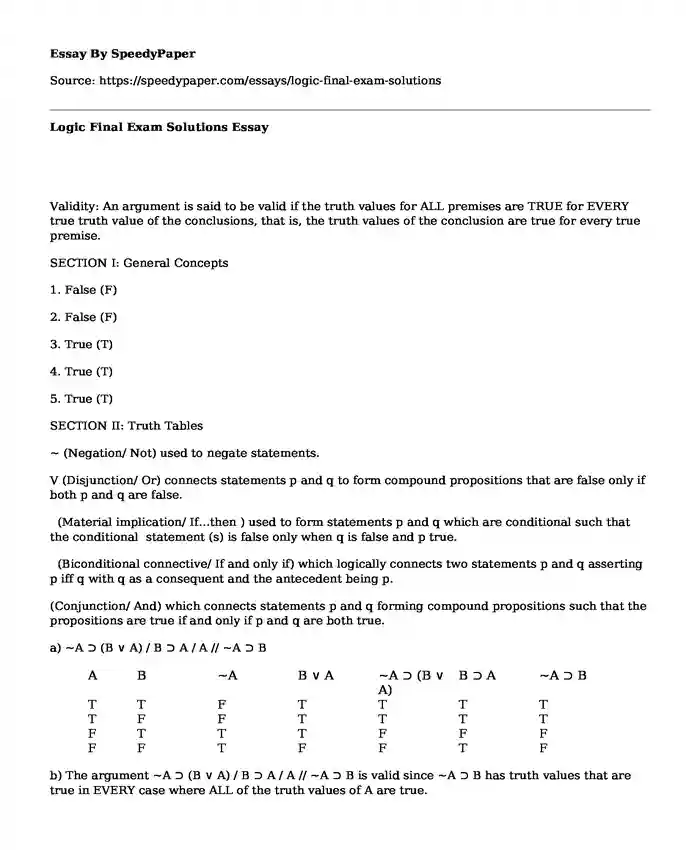# Logic Final Exam Solutions

Published: 2018-01-31Type of paper: Essay Categories: Analysis Mathematics Pages: 3 Wordcount: 644 words
143 views

Validity: An argument is said to be valid if the truth values for ALL premises are TRUE for EVERY true truth value of the conclusions, that is, the truth values of the conclusion are true for every true premise.

Is your time best spent reading someone else’s essay? Get a 100% original essay FROM A CERTIFIED WRITER!

SECTION I: General Concepts

1. False (F)

2. False (F)

3. True (T)

4. True (T)

5. True (T)

SECTION II: Truth Tables

~ (Negation/ Not) used to negate statements.

V (Disjunction/ Or) connects statements p and q to form compound propositions that are false only if both p and q are false.

(Material implication/ If…then ) used to form statements p and q which are conditional such that the conditional statement (s) is false only when q is false and p true.

(Biconditional connective/ If and only if) which logically connects two statements p and q asserting p iff q with q as a consequent and the antecedent being p.

(Conjunction/ And) which connects statements p and q forming compound propositions such that the propositions are true if and only if p and q are both true.

a) ~A ⊃ (B ∨ A) / B ⊃ A / A // ~A ⊃ B

 A B ~A B ∨ A ~A ⊃ (B ∨ A) B ⊃ A ~A ⊃ B T T F T T T T T F F T T T T F T T T F F F F F T F F T F

b) The argument ~A ⊃ (B ∨ A) / B ⊃ A / A // ~A ⊃ B is valid since ~A ⊃ B has truth values that are true in EVERY case where ALL of the truth values of A are true.

SECTION III: Rules of Replacement

1. Duplication 4. Equivalence 7. Commutativity 10.Implication

2. Negation 5. Simplification 8. Conjunction

3. Dilemma 6. Disjunction Introduction 9. Conditional Exchange

SECTION III: Proofs using Rules of Replacement

1.

1.) (1) (~M ⊃ P)(~N ⊃ Q)

2) ~(MN) /∴ P ∨ Q

(3) ~M ∨ ~N 2 Dem

(4) P ∨ Q 2 Imp

2. 2.) (1) R ⊃ ~B

(2) D ∨ R

(3) B /∴ D

(4) ~~B 3DN

(5) ~R 1,2 MT

(6) R ∨ D 2Com

(7) D 2,6DI

3.) (1) ~ ~ ~(O ∨ M)

(2) ~S /∴ ~M

(3) ~(O ∨ M) 1DN

(4) ~O~M 3DeM

(5) ~M~O 4 Com

(6) ~M 2-5 IMP

4.) (1) ~(~E~N) ⊃ T

(2) G ⊃ (N ∨ E) /∴ G ⊃ T

(3) (~~E ∨ ~~N) ⊃ T 1DeM

(4) (E ∨ ~~N) ⊃ T 3DN

(5) (E ∨ N) ⊃ T 4DN

(6) (N ∨ E) ⊃ T 5Com

(7) G ⊃ T 2IMP

SECTION IV: Proofs

a.) 1. M ⊃ (U ⊃ H)

2. (H ∨ ~U) ⊃ F / M ⊃ F

3. H ∨ ~U 2IMP

4. F 2MP

5. H 3SIMP

6. ~U 3SIMP

7. M 1 SIMP

8. M ⊃F

b.) 1. ~H ⊃ B

2. ~H ⊃ D

3. ~(B • D) / H

4. B • D 3IMP

5. B 4SIMP

6. D 4SIMP

7. ~H 1,5MP

8. ~ ~H 7DN

9. H